The radius of a Triangle's Circumcircle or of a Polyhedron's Circumsphere, denoted. For a Triangle,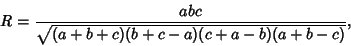(1)

where the side lengths of the Triangle are,, and.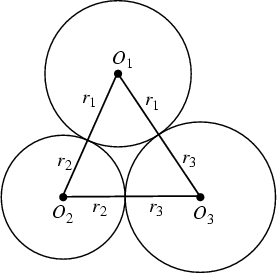This equation can also be expressed in terms of the Radii of the three mutually tangent Circles centered at the Triangle's Vertices. Relabeling the diagram for the Soddy Circles with Vertices,, andand the radii,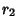, and, and using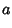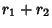(2)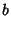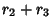(3)(4)

then gives(5)

If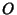is the Circumcenter and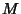is the triangle Centroid, then(6)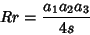(7)(8)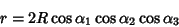(9)(10)

(Johnson 1929, pp. 189-191). Letbe the distance between Inradiusand circumradius,. Then(11)(12)

(Mackay 1886-87). These and many other identities are given in Johnson (1929, pp. 186-190).

For an Archimedean Solid, expressing the circumradius in terms of the Inradiusand Midradiusgives(13)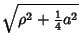(14)

for an Archimedean Solid.

Mackay, J. S. Historical Notes on a Geometrical Theorem and its Developments [18th Century].'' Proc. Edinburgh Math. Soc. 5, 62-78, 1886-1887.